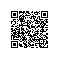# java_数据类型_运算符_200708

 chapter2dir;
/*

java

数值型：
整数型：byte、short、int、long
浮点型：float、double
字符型：char
布尔型：boolean

类：class
接口：interface
数组：array []
*/
public class q1DataType {
public static void main(String[] args){
System.out.println(3+4+"hello"); //7hello
System.out.println("hello"+2+3); //hello23
System.out.println('a'+1+"hello"); //98hello
System.out.println("hello"+'a'+1); //helloa1

char a1 = 97;
System.out.println(a1); //a
int a2 = (int)a1;
System.out.println(a2); //97

// 顺带提一下运算符
int b1 = 2;
int b2 = 5;
double result1 = b2/b1;
System.out.println(result1);    //2.0
double result2 = b2/(double)b1;
System.out.println(result2);    //2.5

//前++(先加再赋值) 后++
int d = 5;
int d1 = ++d;
System.out.println("d = "+d+" d1 = "+d1); //d = 6 d1 = 6
int c = 5;
int c1 = c++;
System.out.println("c = "+c+" c1 = "+c1); //c = 6 c1 = 5

// 赋值运算
short s1 = 10;
// s1 = s1 + 2; //编译不会通过
s1 += 2;    // 不会改变变量本身的数据类型，编译可以通过
System.out.println(s1); // 12

// 逻辑运算符 只有布尔一种数据类型
// $与$\$ 短路与：只要遇到false就直接退出，不执行后面语句。
// ｜ 或      || 短路或：只要遇到true就触发短路。
// ！ 非      ^ 异或：两者不同即为true。

// 位运算符 操作的数据类型是整数值类型 用的比较少
// << 左位移 空位补0，被移除的高位丢弃。   21<<2  结果为84 二进制向左移动用0补。 每向左移动一位，相当于再原来数上乘以2，有限度。
// >> 右位移 高位是0，右移后，空位补0；最高位是1，空位补1。
// >>> u右位移 无论高位0/1，都用0补齐。
// & 只有1&1结果是1，否则0。
// ｜ 只有0｜0结果是0，否则1。
// ^ 1^1为0 0^0为0 1^0为1 0^1为1
// ～ 取反：包括符号位再内所有的0变1，1变0。正数取反，各二进制码按补码各位取反；负数取反，各二进制码按补码各位取反

// 运算符六：三元运算符 (都可以用if-else代替)
// 获取两个整数的较大值
int m = 15;
int n = 2;
int max = (m > n) ? m : n;
System.out.println(max);    // 15
// 升级版
String max2 = (m > n) ? "m大" : ((m == n) ? "一样大" : "n大");
System.out.println(max2);

}
}使用钉钉扫一扫加入圈子
+ 订阅